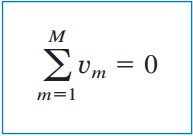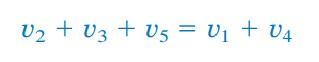omG8HI9LSKFW6lhCj8prTs0Z6lUhdOT9Jhi1Sf4m

# Kirchhoff's Circuit Laws Theory and Equation

Kirchhoff's laws deal with the calculation of current and voltage in electric circuit analysis. This laws summarized the research of Georg Ohm and James Clerk Maxwell. Kirchhoff's laws are flexible because we can use these in frequency and time domain. We need to learn about these fundamental analysis theory.

## Kirchhoff's Laws

Ohm's law alone is not suffiecient to cover the analyzing electric circuit. But, if we pair it with Kirchhoff's two laws, we get a powerful set of analyzing a large variety of electric circuit. Kirchhoff's laws is introduced back when 1824 - 1887 by Gustav Robert Kirchhoff. Thus, these laws are known as Kirchhoff's current laws (KCL) and Kirchhoff's voltage laws (KVL).

## Kirchhoff's Current Law

Kirchhoff's first law is based on the law of conservation of charge that requires that the algebraic sum of charge within a system cannot change. Hence,
Kirchhoff's current laws (KCL) states that the algebraic sum of currents entering a node (or a closed boundary) is zero.
The mathematic equation of KCL is(1)

where :
N   = number of branches which connected to the node
in  = the nth current entering or leaving the node

With this law, currents entering a node can be assumed as positive, while the leaving currents as negative or vice versa.

In order to prove KCL, a set of currents ik(t), k = 1,2,....., flow into a node, the algebraic sum of current at that node is(2)
Integrating both sides of Equation.(2) makes(3)
where qk(t) = ∫ ik(t)dt and qT(t) = ∫ iT(t)dt. But the law of conservation of electric charge requires an algebraic sum of electric charges at the node must not change.
Thus, qT(t) = 0 so that iT(t) = 0, confirming the validity of KCL.
Please give attention to Figure.(1) below, an ilustrated KCL.Figure 1. Kirchhoff current law

Consider the node in Figure.(1). Applying the KCL makes(4)

since the currents i1i3,and i4 are entering the node, while the i2 and i5 are leaving the node, we can arrange the Equation.(4) to(5)

Equation.(5) is an alternative form of KCL:
The sum of currents entering a node is equal to the sum of the currents leaving the node.
Take a note that KCL also applies to a closed boundary. Therefore, this can be generalized case because a node may be assumed as a closed surface shrunk to a point. Figure.(2) is illustrating a two dimensions boundary where the total currents entering the closed surface is equal to the total current leaving the surface.Figure 2. Kirchhoff current law closed boundary

For easier explanation, let us imagine some current sources connected together in parralel. The combined current is the algebraic sum of the current supplied by individual sources. Then, this example can be seen ini Figure.(3a) and then combined to make connection in Figure.(3b).Figure 3. Equivalent example of Kirchhoff current law

The combined or equivalent current source can be found by applying KCL to node a.(6)

A circuit cannot contain two different current I1 and I2 in series unless I1 = I2.

## Kirchhoff's Voltage Law

Kirchhoff's second law is based on the principle of conservation of energy :
Kirchhoff's voltage law (KVL) states that the algebraic sum of all voltages around a closed path (or loop) is zero.
With mathematic equation, KVL states(7)

where M is the number of voltages in the loop (or the number of branches in the loop) and vm is the mth voltage.

For better understanding, please take a look on Figure.(4).Figure 4. Kirchhoff voltage law
The sign on each voltage is the polarity of the terminal encountered first as we travel around the loop. So that, we can start with any branch and go around the loop either clockwise direction or counterclockwise. Assume we start with clockwise direction then the voltages would be -v1, +v2, +v3, -v4, and +v5 in order. Hence the KVL yields(8)
Rearranging equation gives(9)
Which may be interpreted as(10)
For example for the voltage sources in Figure.(5a)Figure 5. Kirchhoff voltage law equivalent circuit
the combined or equivalent voltage source in Figure.(5b) is obtained by using KVL.(11)
Using two differerent voltages in parallel is violating KVL unless the values are the same.

## Kirchhoff's Laws Examples

1.For circuit in Figure.(6), find voltages v1 and v2.Figure 6

Solution :
To find v1 and v2, we apply Ohm's law and Kirchhoff's voltage law.
Assume the current is flowing in clockwise direction.
From Ohm's law,
v1 = 2iv2 = -3i..............(1.1)
Applying KVL
-20 + v1 v2 = 0..............(1.2)
Substituting equation (1.1) and (1.2) above, we get
-20 + 2i + 3i = 0
or
5i = 20
then
i = 4 A
Substituting i in (1.1) we get

v1 = 8 V and v= - 12 V

2.Determine vo and i in Figure.(7)Figure 7

Solution :
We apply KVL around the loop, gives
- 12 + 4i + 2vo - 4 + 6i = 0............(2.1)
Applying Ohm's law to 6Ω gives
vo = -6i...........(2.2)
Substituting (2.1) and (2.2) gives
-16 + 10i - 12i = 0 => i = -8 A
and
vo = 48 V

## Kirchhoff's Voltage Law Calculator

After learning about Kirchhoff's laws, we can use a simple KVL calculator below to make our work easier.

## Kirchhoff's Current Law Calculator

After learning about Kirchhoff's laws, we can use a simple KCL calculator below to make our work easier.

Untuk Bahasa Indonesia baca Hukum Kirchhoff : Kuasai Hukum Dasar dan Mudah Bagi Teknik Elektro
Related Posts
SHARE